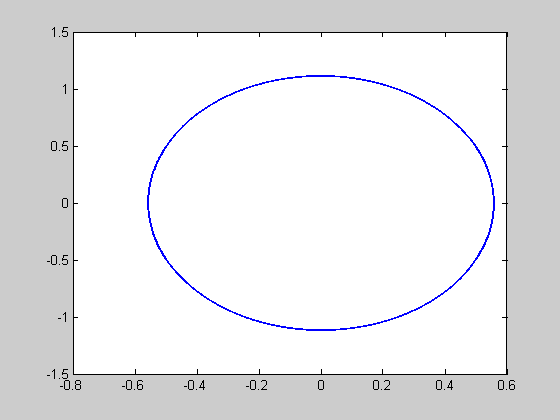# Calculation and plot of the poincare section of two uncoupled osciators with omega1 and omega2 : omega1/omega2=p/q=rational -> closed orbit, periodic behaviour; omega1/omega2=irational -> quasiperiodic behaviour

## Contents

```close;
clear;
ps=zeros(2,1);
```

## integration of torus.m

```[t,y]=ode45(@torus,[0:0.001:100],[0.5 0.5 0.5]);
%plot projection of the phas space orbit to y1-y2 plane
plot(y(:,1),y(:,2));
hold;
```
```Current plot held
```## calculation of poincare section

calculate number of integration steps

```n=size(t);
%set the index of poincare points to 1
np=1;
for i=1:n(1)
% detect the cros-section of the trajectory with the plane y1-y2
if(y(i,3)>=(2*pi)*np)
% store detected cross-section point y1,y2 to ps1,ps2
ps(np,1)=y(i,1);
ps(np,2)=y(i,2);
% increase the index of poincare point
np=np+1;
end
end
```

## plot the poincare section

```for i=1:np-1
plot(ps(i,1),ps(i,2),'r+')
% use pause to folow the plot of the poincare section
%pause(2);
end
%plot(ps(:,1),ps(:,2),'r+');
```## torus.m file - two uncoupled oscilators with omega1=2 and omega2=3

```%function dy=torus(t,y)
%dy=zeros(3,1);
%dy(1)=y(2);
%dy(2)=-(omega1)*y(1);
%dy(3)=omega2;
```